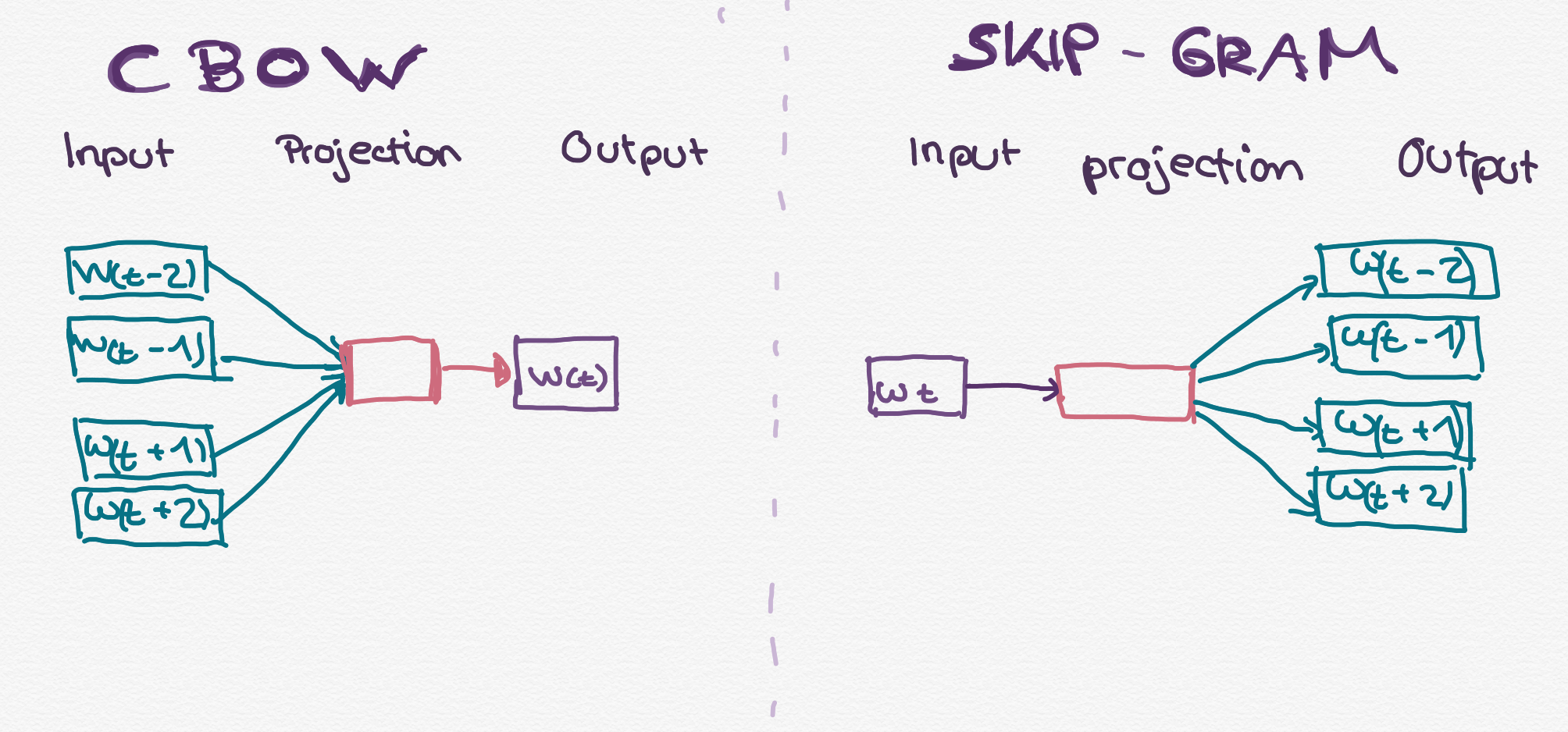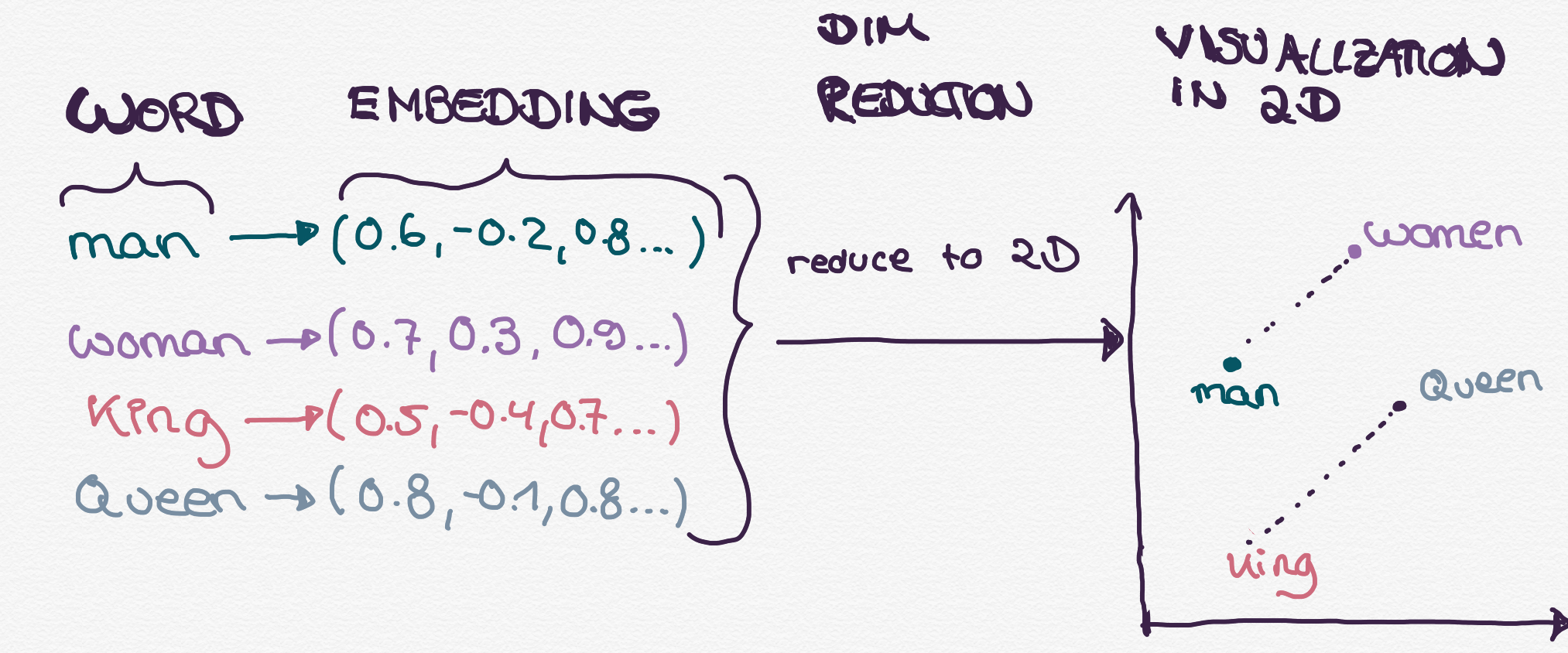Humans intuitively understand the meaning of words: Which words are similar, opposites or related to each other? But our machine learning models do not have this intuition. Word embeddings are numeric vectors that represent text. These vectors are learned through neural networks. The objective when creating these embedding vectors is to capture as much “meaning” as possible: Related words should be closer together than unrelated words. Also, they should be able to preserve mathematical relationships between words such as

king — man = queen — woman

france - paris = italy - rome.

In this blogpost, we will start with (probably) the simplest way to numerically represent text: One-hot-encoding. Then we will look into two famous word embedding models: Word2Vec and BERT and learn which kind of relationships between words are captured.

## What’s wrong with just using One-Hot-Encoding (a.k.a. Dummy variables)?

The easiest way to encode words is through one One-Hot encoded vectors: Each unique word or token has a unique dimension and will be represented with a 1 if present and with 0s everywhere else. Your result is a huge and sparse matrix with 0s and 1s. Let’s take two sentences

• s1: “I am sitting on the river bank.”
• s2: “I am going to the bank to get some money”.

The two sentences share some common words (“I”, “am”, “the” and “bank”). The word “bank”, however, has a different meaning in both sentences. If you use One-Hot-Encoding you get the following matrix:

I am going to the river bank get some money
s1 1 1 1 1 1 1 1 0 0 0
s2 1 1 1 1 1 0 1 0 1 1

The larger the text, the larger the matrix. If you applied One-Hot-Encoding to fulltext data you would get larger, sparser matrices. So One-Hot-Encoding may make sense, if you have a limited number of words in your text (e.g. if you want to vectorize gender or a profession from a text field).

In addition, it disregards how many times a word appears in a sentence and therefore does not capture the importance of words. Every word will always get a value between 0 or 1. For considering the importance of words, a TF-IDF-Measure transformation can be applied. By weighting words with their term frequency divided by their inverse document frequency, rare words that appear a lot in one sentence are weighted more than words that appear a lot in every sentence and in many sentences (e. g. stopwords such as “the”, “a”, “or”).

But there would still be two major drawbacks: You cannot differentiate between a river bank and the bank, where you store your money. And you cannot grasp the relationship between words: Are words similar? Are they somehow related?

## Word2Vec

Word2vec is a three-layer neural network in which the first is the input layer and the last layers are the output layer. Input and output layers are one-hot-encoded vectors of the text. The middle layer builds a latent representation, so the input words are transformed into the output vector representation.

• inputs represented as one-hot vectors
• outputs represented as one hot vectors (in training)
• embedding layer: matrix of all the words found in the vocabulary and their embedding (some numeric value that isinitialized randomly)[vocab size x embedding size]

Continuous Bag of Words (CBOW) predicts a word given its context. In other words: it learns an embedding by predicting a word by using the surrounding words it as features.

Continous Skip Gram predicts the context having one target word as the input. It learns an embedding by predicting the context by using the words as features.If you want to retrain or train a Word2Vec Model, you have to define a context, the number of words appearing on the left and right of a focus word. If you choose for example 3, then the context would include the 3 words before and the 3 words after the focus word. Note that this approach does not consider the order of words. Either a word is in the context or it is not. Word2Vec then uses a stochastic optimizer to train the neural network.

The creators evaluated the model by marking sure similar words have similar vectors. You can visualize similarity by analyzing cosine similarity or by plotting the embeddings from the original models, e.g. https://projector.tensorflow.org.

Word2Vec registers relations among words. So if you subtract the man from king you get the same as when you subtract the women from queen. Great, this model is able to retain mathematical relationships between words.If you check for the five most similar words in terms of cosine similarity for “bank”, you get “banks”, “monetary”, “banking”, “financial” and “trade”. So the model seems to primarily interpret “bank” in the sense of a financial institute. Don’t forget the model is trained on news data, so if you apply it to very specific contexts (e.g. scientific domain data), you may lack specific words and relations. The word “bank” will always have the same numeric representation. So the model fails to understand the difference between the river bank and the financial institute bank.
If you wanted to use Word2Vec on text data about river banks, you would have to retrain the model.

## BERT

One embedding model, that became pretty popular and that was considered as a great break-through, is BERT. BERT produces word representations that are dynamically informed by the words around them. That means: BERT understands that the word “bank” can mean something different depending on the context.

So what is exactly BERT? BERT stands for Bidirectional Encoder Representations from Transformers. Let’s break that up: Bidirectional means, that instead of going sequentially through the words, it goes from left to right and from right to left. It uses the transformer logic (a special type of neural net that contains two sublayers and a fully connected feed forward network) to encode representations of words. There are two English versions of BERT: A smaller base version and a large Version. The larger version has double the amount of encoders and attention heads and more than 3 times as many parameters

nr Encoders nr biderectional self-attention heads nr parameters
BERT base 12 12 110
BERT large 24 24 340

BERT follows a 2-step process: pre-training and fine-tuning

1. Pre-Train a language model on a large unlabelled text corpus (data extracted from BooksCorpus (800M words) and English Wikipedia (2,500M words)) by using a masked language model and next sentence prediction. This type of training is novel as it is an unsupervised approach that can be easily transferred to other datasets without manually labeling data.

2. Fine-tune the model. You can finetune the model with a layer of untrained neurons as a feedforward layer on top of the pre-trained BERT for specific domain knowledge (But you could also add a supervised additional output layer to solve for example classification tasks or build chatbots, which makes the model really powerful)

## Summary

We have seen three approaches on how to create numeric representations of text. Let’s briefly recap, what the different approaches are helpful for:

One-Hot-Encoding Word2Vec BERT
relationships between words no yes yes
consider the context of a word no no yes

There exists many more and there are also numerous extensions for Word2Vec and BERT.